﻿ Multi-Factorial Analysis of Atmospheric Noise Pollution Level Based on Emitted Carbon and Heat Radiation during Gas Flaring

### Multi-Factorial Analysis of Atmospheric Noise Pollution Level Based on Emitted Carbon and Heat Radia...

C. I. Nwoye, S. O. Nwakpa, I. E. Nwosu, J. U Odo, E. C. Chinwuko, N. E. IdenyiOPEN ACCESSPEER-REVIEWED

## Multi-Factorial Analysis of Atmospheric Noise Pollution Level Based on Emitted Carbon and Heat Radiation during Gas Flaring

C. I. Nwoye1,, S. O. Nwakpa1, I. E. Nwosu2, J. U Odo1, E. C. Chinwuko3, N. E. Idenyi4

1Department of Metallurgical and Materials Engineering, Nnamdi Azikiwe University, Awka, Nigeria

2Department of Environmental Technology, Federal University of Technology, Owerri, Nigeria

3Department of Industrial and Production Engineering, Nnamdi Azikiwe University, Awka, Nigeria

4Department of Industrial Physics Ebonyi State University, Abakiliki, Nigeria

### Abstract

This paper presents a multi-factorial analysis of atmospheric noise pollution level based on emitted carbon and heat radiation during gas flaring. An empirical model; three factorial in nature was derived, validated and used for the noise pollution level analysis. The derived model showed that the noise pollution level was basically dependent on gas flaring output parameters such as emitted carbon and heat radiation since the three occur at the same time, and also on reference distance from flare point, total associated gas and total gas produced. The validity of the model; ϑ=D Log ϕ[Log ϕ (0.0001ζ2+ζ)+₰]-1 was rooted on the core model expression D / ϑ ≈ S ζ2 + ζ + (₰ / Log ϕ) where both sides of the expression are correspondingly approximately equal. Regression model was used to generate results of noise pollution level, and its trend of distribution was compared with that from derived model as a way of verifying its validity relative to experimental results. The results of this verification translated into very close alignment of curves, dimensions of shapes and areas covered. These translated into significantly similar trend of data point’s distribution for experimental (ExD), derived model (MoD) and regression model-predicted (ReG) results. Evaluations from generated results indicated that noise pollution level per unit radiated heat & emitted carbon as obtained from experiment and derived model were 60.42 and 60.00 dBA / Kw m-2 & 4.01 x10-4 and 3.98x10-4 dBA /ton respectively. Standard errors incurred in predicting noise pollution level for each value of the radiated heat, emitted carbon & Total associated gas/ Total gas produced; TAG/TGP as obtained from experiment and derived model were 6.6533 and 5.7521%, 6.6405 and 3.1291 % & 6.6616 and 3.9963% respectively. The least and highest deviation of model-predicted noise pollution level (from experimental results) were 1.62 and 21.42%, implying a model operational confidence level range 78-98%.

### At a glance: Figures

12345
Prev Next

• Nwoye, C. I., et al. "Multi-Factorial Analysis of Atmospheric Noise Pollution Level Based on Emitted Carbon and Heat Radiation during Gas Flaring." Journal of Atmospheric Pollution 2.1 (2014): 22-29.
• Nwoye, C. I. , Nwakpa, S. O. , Nwosu, I. E. , Odo, J. U. , Chinwuko, E. C. , & Idenyi, N. E. (2014). Multi-Factorial Analysis of Atmospheric Noise Pollution Level Based on Emitted Carbon and Heat Radiation during Gas Flaring. Journal of Atmospheric Pollution, 2(1), 22-29.
• Nwoye, C. I., S. O. Nwakpa, I. E. Nwosu, J. U Odo, E. C. Chinwuko, and N. E. Idenyi. "Multi-Factorial Analysis of Atmospheric Noise Pollution Level Based on Emitted Carbon and Heat Radiation during Gas Flaring." Journal of Atmospheric Pollution 2, no. 1 (2014): 22-29.

 Import into BibTeX Import into EndNote Import into RefMan Import into RefWorks

### 1. Introduction

The growing need for safety and prevention of explosion due to piled-up gas pressure from oil chemical producing operations has resulted to the design and development of flare systems to relieve emergency process upsets that require release of large volumes of gas. These flare systems have been technically described in many papers (Bader et al. 2011; Hong et al. 2006). In the course of routine oil and gas production, flaring operations are basically, controlled burning of gases, in open flames and open air. Venting, on the contrary, is the controlled release of gases into the atmosphere, without combustion.

The traditional method for the safe disposal of large quantities of unwanted flammable gases and vapours in the oil industry has remained combustion of these gases (Brzustowski, 1976; Dubnowski and Davis, 1983). Research (API, 1990) has shown that application of combustion basically for conversion of flammable, toxic or corrosive vapors to less objectionable compounds is the primary function of a flare. Report (AEUB, 1999) has shown that about 70% of the total gas flared has been converted to solution gas in Alberta, implying that the gas was separated from produced oil or bitumen.

Generation of noise, heat radiation, and emission of atmospheric pollutants have been observed (Obia et al., 2011) to occur as flare operates.

On having a good mixing between the fuel gas and air, implying efficient combustion, the emitted gases are mainly water vapour and carbon dioxide. Other pollutants such as carbon monoxide (CO), nitrogen oxides (NOX), sulphur dioxide (SO2), volatile organic compounds (VOC) and particulate matter (PM) are generally present, even if the combustion efficiency is higher than 90%. VOCs are derived by incomplete combustion of the flared gas, or by its conversion to other compounds, such as aldehydes or acids and could be eliminated to near completion, exceeding 98%. Smoke formation, most probably occurs in streams with high carbon/hydrogen mole ratio (greater than 0.35).

In the industries, gas flaring would have been so advantageous to avoid, but such operation is necessary under different circumstances as, for example: (1) to release unburned process gas from the processing facilities (2) to release high pressure in the plant and avoid catastrophic situation (3) to burn vapours collected from the tops of tanks as they are being filled (4) to eliminate the excess gas which cannot be supplied (5) after process upset, equipment change over or maintenance.

Basically, two types of flares predominate in industry: the ground flare and the elevated flare. Ground flares are primarily designed for low release rates and are not effective for emergency releases. In elevated flares, the stack heights are up to 400ft with diameters over 40 inches. The high elevation reduces potential flaring hazards because ground level radiation is lower and better dispersion of gases occurs should the flame be snuffed out (Dubnowski and Davis, 1983).

Both flares can further be classified according to the method used for enhancing mixing between air and fuel. In the steam-assisted flares steam is injected into the combustion zone to promote turbulence and entrain air into the flame. In the air-assisted flares forced air is used to provide the combustion air and mixing; these flares can be used when steam is not available. The non-assisted flares do not have any mechanism for enhancing the air-flame mixing; they are used for gases with a low heat content and a low carbon/hydrogen ratio (such ratio is related to the smoke production). Finally, the pressure-assisted flares use the vent stream pressure to promote mixing between air and flame.

Calculation of radiation level K (Kw/m2) at distance d (m) has been performed API (2007) and EPA (1995) assuming the flame as a single radiant point located at its centre, using the following equation: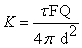(1)

where F is the fraction of heat released which is radiated (-), Q is the heat liberated (kW) obtained by multiplying the mass flow rate (kg/h) by the heat of combustion (kJ/kg), and τ is the transmissivity (-), which is the fraction of heat radiated transmitted through the atmosphere. The transmissivity basically depends on how the atmosphere absorbs radiated heat. Since water vapour is the most important absorber in air, relative humidity will play an important role. Transmissivity is calculated using an empirical equation derived by Brzustowski and Sommer (1973)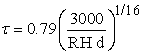(2)

where RH is the relative humidity (%).

Results evaluation (Odigure et al., 2003) has shown that heat radiation from the flare station is a function of the flare temperature, gas flow rate and the geometrical design of flare stack.

An intensive study (Cooke et al., 1987b) has shown that the fraction of radiated heat expresses the total radiant power output of a flare as a fraction of the total chemical power input. This dimensionless number allows for the fact that not all of the heat released in a flame can be transferred by radiation (API, 1990). The fraction of heat radiated is an overall characteristic of the flame, which can be affected by the following variables (Schwartz and White, 1996): (1) flame temperature (2) gas composition (3) soot/smoke formation (4) flame type (5) flare burner design (6) state of air-fuel mixing (7) quantity of fuel being burned.

The fraction of heat radiated has been referred to in the literature as the F-factor, F and Fs (API, 1990; Cooke et al., 1987; Leahey and Davies, 1984; McMurray, 1982; Chamberlain, 1987).

It has been reported (Chapra, 1997) that the models and relationships of the fraction of radiated heat are divided into categories: theoretical relationships, which are based on a deductive or theoretical approach; involving the use of mechanistic relationships or organizing principles. Empirical relationships are based on an inductive or data-based approach. Regression methods are often employed to statistically estimate the relationships between parameters

A theoretical relationship between the fraction of radiated heat and the molecular weight of the gas was proposed by Tan (1967). Tan derived the following equation for the fraction of radiated heat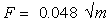(3)

Where m = molecular weight of the flared gas.

Section 521 of The American Petroleum Institute Recommended Practice (API, 1969) provides a theoretical equation for calculating the fraction of heat radiated F at a minimum distance D from a flare to an object whose exposure must be limited as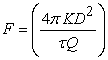(4)

Where

D = minimum distance from the midpoint of the flame to the object being considered, in feet

τ = fraction of heat intensity transmitted

F = fraction of heat radiated

Q= net heat release (lower heating value), in British thermal units per hour (kilowatts)

K= allowable radiation, in British thermal units per hour per square foot (kilowatts per square meter)

Report (Chamberlain, 1987) idealized the flame as a frustum of a cone, and defined the fraction Fs of the net heat content of the flame that appears as radiation from the surface of this solid body in terms of surface emissive power. Following this analysis, the researcher gives a theoretical relationship between fraction of heat radiated from surface of flame and other variables as: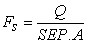(5)

Where

SEP = surface emissive power (kW/m2)

Fs = fraction of heat radiated from surface of flame

A = surface area of frustum including end discs (m2)

Q = net heat release (kW)

Further work (Chamberlain, 1987) established an empirical relationship between fraction of radiated heat and gas exit velocity The model expressed as;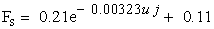(6)

was also applied in describing the correlation between both parameters.

Where

uj = gas velocity (m/s)

Fs = fraction of heat radiated from flame surface

Another researcher (Cook et al., 1987) provided the following empirical correlation between fraction of heat radiated and the jet exit velocity: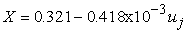(7)

Where

X = fraction of heat radiated

uj = jet exit velocity (m/s)

It was found that over 80% of all predictions were within 30% of the measurements.

The aim of this work is to carry out a multi-factorial analysis of atmospheric noise pollution level based on emitted carbon and heat radiation during gas flaring. The work is stemmed on the fact that flare generates noise, heat radiation, and emits atmospheric pollutants as it operates (Obia et al., 2011).

### 2. Noise level Predictive-Model (NOPMOD)

#### Table 3. Variation of D/ ϑ with 0.0001 ζ2 + ζ + (₰ /Log ϕ)

In order to under score the dependence of noise pollution level on some process parameters, during the gas flaring process, a model will be derived, validated and pplied for easy analysis.

2.1. Model formulation

Computational analysis (using C-NIKBRAN: Nwoye, 2008) of results in Table 1 indicates that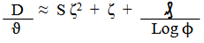(8)

Introducing the value of S into equation (8) reduces it to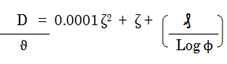(9)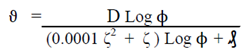(10)

Where

S = Empirical constant; 0.0001

(ϑ) = Noise level (dBA)

(₰) = Radiated heat from the flare (Kw/m2)

(ζ) = Total associated gas/ Total gas produced

(ϕ) = Emitted carbon (Tonne)

TAG= Total non-associated gas (Mscf/d)

TGP = Total gas produced (Mscf/d)

D = Reference distance from flare point (m)

During the model development, a soft ware; C-NIKBRAN was used to map-link the various parameters such emitted carbon ϕ, radiated heat ₰, total associated gas - total gas produced ratio ζ, and reference distance D, from flare point with the view to establishing an empirical relationship between noise level ϑ and the other parameters. Map-linking of the experimental or field work results were carried out using Table 1 and Table 2, which contain the field work out put parameters. The result of the data mapping is the core model expression in equation (8), which on evaluation gives Table 3.

### 3. Boundary and Initial Conditions

The ranges of carbon emitted, total associated gas, total gas produced, radiated heat and noise level are 26.82-14875.66 tonnes, 781 – 863803.8 Mscf/d, 781 -1184054.5, 0.283 – 0.657 and 63.1 – 69.8 respectively. The distance D from the flare point = 75m. The flow rate of the gas was assumed constant.

### 4. Results and Discussion

4.1. Model Validation

Model validation was carried out using predicted results generated at 75m as the reference distance D from the flare point. It was only at this distance that predicted heat radiation levels were in significant proximate agreement with experimental results, indicating model validity. Preliminary mathematical evaluations show that D values less and greater than 75m predicted heat radiation levels that completely disagreed with experimental results in terms of proximity. The derived model was rooted in equation (8). Equation (8) agrees with Table 4 following the values of D/ ϑ and S ζ2 + ζ + (₰ /Log ϕ) and evaluated from Table 1 and Table 2.

Emitted carbon ϕ, radiated heat ₰, total associated gas - total gas produced ratio ζ, and reference distance D, from flare point are all output parameters (except D) generated during field work/ experiment (Onyejekwe, 2012) in the course of studying the flaring process. The empirical model derived for analysis of the atmospheric noise pollution ϑ, relates ϑ with the other parameters such that noise pollution level in flaring sites could be determined (as model out-put) at any point in time without further field work by substituting the highlighted output parameters (now as input parameters) into the derived model, providing these input parameters are within the range of values generated during the initial field work under the initial field work conditions (Boundary and initial conditions).

Based on the forgoing, substitution of the model input parameters into the derived model, outside these boundary and initial conditions: emitted carbon 26.82-14875.66 tonnes, total associated gas: 781 – 863803.8 Mscf/d, total gas produced: 781 - 1184054.5 Mscf/d, radiated heat 0.283 – 0.657 (Kw/m2) and distance D (from the flare point): 75m, makes the predicted noise pollution level unreliable. The initial condition is rooted on the condition that the flow rate of the gas was constant.

The limitation of the derived model is that it cannot operate outside the highlighted boundary and initial condition of the initial field work. The derived model could be improved on by adding a correction factor to the derived model expression so as to take care of the prevailing field work conditions, which play vital roles during the field work, but were not considered during model formulation.

Furthermore, the derived model was validated by comparing the noise level predicted by the model and that obtained from the experiment (Onyejekwe, 2012). This was done using the 4th Degree Model Validity Test Techniques (4th DMVTT); computational, graphical, statistical and deviational analysis (Nwoye and Nwabanne, 2013).

4.2. Statistical Analysis
4.2.1. Standard Error (STEYX)

The standard errors in predicting the noise pollution level (using experimental and model-predicted results) for each value of the quantity of radiated heat, emitted carbon & TAG/TGP are 6.6533, 6.6405 & 6.6616% and 5.7521, 3.1391 & 3.9963 % respectively. The standard error was evaluated using Microsoft Excel version 2003.

4.2.2. Correlation (CORREL)

The correlation coefficient between noise pollution level and quantity of radiated heat, emitted carbon as well as the operational ratio TAG/TGP were calculated from the results of derived model and experiment. This was done by considering the coefficients of determination R2 from Figures 2-7 and then evaluating them using the expression: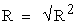(11)

The evaluated correlations (using Microsoft Excel version 2003) are shown in Tables 4-6. These evaluated results indicate that the derived model predictions are in proximate agreement between actual experimental and model-predicted results.

#### Table 6. Comparison of the correlations evaluated from derived model predicted and ExD results based on TAG/TGP

4.3. Graphical Analysis

Critical comparative analysis of Figures 8-10 shows close alignment of the curves and dimensions of shapes and areas covered by model-predicted noise pollution level (MoD) (relative to radiated heat, emitted carbon and the ratio TAG/TGP) and those from the experiment (ExD).

Figure 8. Comparison of the noise pollution levels (relative to quantities of heat radiated) as obtained from experiment and derived model

Figure 8- Figure 10 strongly indicate that the degree of alignment of curves and dimension equality of shapes and areas covered at noise levels 66.7, 63.1, 69.6 and 69.8 (Figure 8); emitted carbons 486.39, 26.82, 7719.93 and 117.65 (Figure 9) as well as ratios (TAG/TGP) 1,1,1 and 1(Figure10) is indicative of the proximate agreement between both experimental and model-predicted values noise level. This also indicates that the derived model is valid, reliable and exhumes a very high operation confidence.

Figure 9. Comparison of the noise pollution levels (relative to emitted carbon) as obtained from experiment and derived model
Figure 10. Comparison of the noise pollution levels (relative to the ratio TAG/TGP) as obtained from experiment and derived model

4.3.1. Comparison of Derived Model with Standard Model

The validity of the derived model was further verified through application of the regression model (ReG) in predicting the trend of the experimental results. Critical comparative analysis of Figures 8-13 shows very close alignment of curves, dimensions of shapes and areas covered. These translated into significantly similar trend of data points distribution for experimental (ExD), derived model-predicted (MoD) and regression model (ReG) predicted results also at noise levels 66.7, 63.1, 69.6 and 69.8 (Figure 8); emitted carbons 486.39, 26.82, 7719.93 and 117.65 (Figure 9) as well as ratios (TAG/TGP) 1,1,1 and 1 (Figure 10).

Figure 11. Comparison of the noise pollution levels (relative to quantities of heat radiated) as obtained from experiment, derived model and regression model
Figure 12. Comparison of the noise pollution levels (relative to emitted carbon) as obtained from experiment, derived model and regression model

Furthermore, the standard errors incurred in predicting the noise pollution levels for each value of radiated heat, emitted carbon & TAG/TGP for regression model-predicted results (which translated into ReG shapes and areas in Figure 11- Figure 13) are 2.2426 x 10-5, 0.0009 & 6.2684 x 10-9 respectively. These values are in proximate agreement with both experimental and derived model-predicted results.

Figure 13. Comparison of the noise pollution levels (relative to TAG/TGP) as obtained from experiment, derived model and regression model.
4.4. Computational Analysis
4.4.1. Noise Pollution Level per unit Radiated Heat

The noise pollution level per unit radiated heat ϑR (dBA / Kw m-2) was calculated from the equation;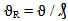(12)

Re-written as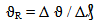(13)

Equation (13) is detailed as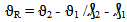(14)

Where

Δϑ = Change in the noise pollution levels ϑ2, ϑ1 at radiated heat values ₰2, ₰1.

Considering the points (0.283, 66.7) & (0.235, 69.6) and (0.283, 67.85) & (0.235, 70.73) as shown in Figure 8, designating them as (ϑ1, ₰1) & (ϑ2, ₰2) for experimental and model-predicted results, and then substituting them into equation (14), gives the slopes: - 60.42 and - 60.00 dBA / Kw m-2 respectively as their corresponding noise pollution level per unit radiated heat.

4.4.2. Noise Pollution Level per unit Emitted Carbon

Similarly, substituting into equation (14) points (486.39, 66.7) & (7719.93, 69.6) and (486.39, 67.85) & (7719.93,70.73) culled from Table 1 and Figure 10, as (ϑ1, ϕ1) & (ϑ2, ϕ2) for experimental and model-predicted results also gives the slopes: 4.01 x10-4 and 3.98x10-4 dBA /ton respectively as their corresponding noise pollution level per unit emitted carbon. The proximity between values in each result set indicates significantly high validity level for the derived model.

It is very pertinent to state that the actual noise pollution level per unit radiated heat (as obtained from experiment and derived model) was just the magnitude of the signed value. The associated sign preceding these values as evaluated signifies that the associated slope tilted to negative plane. Based on the foregoing, the noise pollution level per unit radiated heat as obtained from experiment and derived model were 60.42 and 60.00 dBA / Kw m-2 respectively.

4.5. Deviational Analysis

A comparative analysis of noise pollution levels as obtained from experiment and derived model reveal deviation of model-predicted values from those of the experiment. This is believed to be due to the fact that some considered assumptions and experiment-oriented conditions which prevailed during the actual field work were not considered during the model formulation. This necessitated the introduction of correction factor, to bring the model-predicted values to those of the experimental.

Deviation (De) (%) of the model-predicted noise level from that of the experiment is given by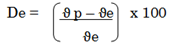(15)

Where

ϑp = Model-predicted noise pollution level (dBA)

ϑe = Noise pollution level evaluated from experiment ( dBA)

Correction factor (Cr) is the negative of the deviation i.e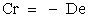(16)

Therefore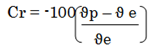(17)

Introduction of the corresponding values of Cf from equation (17) into the model gives exactly the corresponding experimental values.

Figure 14. Variation of model-predicted quantity of radiated heat (relative to noise level) with associated deviation from experiment.
Figure 15. Variation of model-predicted quantity of radiated heat (relative to emitted carbon) with associated deviation from experiment.

#### Table 7. Variation of correction factor to model-predicted noise pollution level with TAG /TGP

Figures 14 and 15 show that the least and highest deviations of model-predicted results (from experimental results) are 1.62 and 21.42 %. These deviations correspond to model-predicted noise pollution levels: 70.73 and 84.51 dBA; quantities heat radiations: 0.235 and 0.657 Kw/m2; emitted carbon: 7719.93 and 14875.66 tonnes; and TAG /TGP: 1 and 0.73 respectively.

Equations (16) and (17) show that correction factor is the negative of the deviation. It is strongly believed that the correction factor takes care of the assumptions made and experimental condition prevailing during the field works which were not considered during the model formulation.

Table 7 indicates that the least and highest correction factor to the model-predicted noise pollution level are -1.62 and -21.42 %. These correction factors correspond to model-predicted noise pollution levels: 70.73 and 84.51 dBA; quantities heat radiations: 0.235 and 0.657 Kw/m2; emitted carbon: 7719.93 and 14875.66 tonnes; and TAG /TGP: 1 and 0.73 respectively.

It is important to state that the deviation of model predicted results from that of the experiment is just the magnitude of the value. The associated sign preceding the value signifies that the deviation is deficit (negative sign) or surplus (positive sign).

### 5. Conclusion

Following an extensive multi-factorial analysis of atmospheric noise pollution level based on emitted carbon and heat radiation (during gas flaring), using an empirical model, the noise pollution level was dependent on gas flaring output parameters such as emitted carbon and heat radiation since the three occur at the same time, and also on reference distance from flare point, total associated gas and total gas produced. The validity of the model was rooted on the core model expression D / ϑ ≈ S ζ2 + ζ + (₰ / Log ϕ) where both sides of the expression are correspondingly approximately equal. Regression model used to generate results of noise pollution level, results of this verification translated into very close alignment of curves, dimensions of shapes and areas covered. These translated into significantly similar trend of data point’s distribution for experimental (ExD), derived model (MoD) and regression model-predicted (ReG) results. Evaluations from generated results indicated that noise pollution level per unit radiated heat & emitted carbon as obtained from experiment and derived model were 60.42 and 60.00 dBA / Kw m-2 & 4.01 x10-4 and 3.98x10-4 dBA /ton respectively. Standard errors incurred in predicting noise pollution level for each value of the radiated heat, emitted carbon & Total associated gas/ Total gas produced; TAG/TGP as obtained from experiment and derived model were 6.6533 and 5.7521%, 6.6405 and 3.1291 % & 6.6616 and 3.9963% respectively. The least and highest deviation of model-predicted noise pollution level (from experimental results) were 1.62 and 21.42%, implying a model operational confidence level range 78-98%.

### References

  Alberta Energy and Utilities Board (AEUB) (1999). ‘Upstream Petroleum Industry Flaring Guide’, Guide Number 60.In article  API (1969). ‘Guide for Pressure-Relieving and Depressuring Systems – American Petroleum Institute Recommended Practice 521’.Washington, D.C.: American Petroleum Institute, Edition 1.In article  API (2007) Standard 521 Pressure-relieving and Depressuring Systems, Fifth Edition, January.In article  API (1990). ‘Guide for Pressure-Relieving and Depressuring Systems – American Petroleum Institute Recommended Practice 521’.Washington, D.C.: American Petroleum Institute, Edition 3.In article  Bader A., Baukal C.E. Jr and Bussman (2011) Selecting the proper flares systems. Chemical Engineering Progress (AIChE). pp. 45-50.In article  Brzustowski T.A. and Sommer E.C. Jr. (1973) Predicting Radiant Heating from Flares. Proceedings-Division of Refining, API 1973, Vol. 53, API Washington, D. C., p. 865-893.In article  Brzustowski, T. A. (1976). ‘Flaring In The Energy Industry’, Progress in Energy and Combustion Science, Volume 2, p129-144.In article CrossRef  Chamberlain, G. A. (1987). ‘Developments in Design Methods for Predicting Thermal Radiation from Flares’, Chemical Engineering, Research and Design, Volume 65.In article  Chapra, S. C. (1997). Surface Water-Quality Modeling, McGraw-Hill Series in Water Resources and Environmental Engineering.In article  Cook, D. K., Fairweather, M., Hammonds, J. and Hughes, D. J. (1987) ‘Size and Radiative Characteristics of Natural Gas Flares. Part 2-Empirical Model’, Chemical Engineering, Research and Design, Volume 65, pp. 310-317.In article  Dubnowski, J. J. and Davies, B. C. (1983). ‘Flaring Combustion Efficiency: A Review of the State of Current Knowledge’, Proceedings of the Annual Meeting Air Pollution Control Association, Atlanta, Georgia, 76th, Volume 4, Published by APCA, Pittsburgh, Pa, USA 83-52. 10, 27p.In article  EPA (1995) SCREEN3 Model User’s Guide. EPA-454/B-95-004. pp. 60.In article  Hong J., Baukal C., Schwartz R and Fleifil M. (2006) Industrial scale flare testing. Chemical Engineering Progress (AIChE). pp. 35-39.In article  Leahey, D. M., and Davies, M. J. E. (1984). ‘Observations of Plume Rise from Sour Gas Flares’, Atmospheric Environment, Volume 18, Number 5, pp. 917-922.In article CrossRef  McMurray, R. (1982). ‘Flare Radiation Estimated’, Hydrocarbon Processing, November 1982, p175-181.In article  Nwoye, C. I. (2008). Data Analytical Memory; C-NIKBRAN Nwoye, C. I. and J. T. Nwabanne. (2013). Empirical Analysis of Methane Gas Yield Dependence on Organic Loading Rate during Microbial Treatment of Fruit Wastes in Digester. Advances in Applied Science Research, 4 (1): 308-318.In article  Obia A.E., Okon H.E., Ekum S.A., Eyo-Ita1 E.E., Ekpeni E.A. (2011) The Influence of Gas Flare Particulates and Rainfall on the Corrosion of Galvanized Steel Roofs in the Niger Delta, Nigeria. Journal of Environmental Protection, 2011, 2, 1341-1346.In article CrossRef  Odigure, J.O.; Abdulkareem, A.S. & O.D Adeniyi, O.D (2003). Computer simulation of soil temperature due to heat radiation from gas flaring. Association for the advancement of Modelling and simulation in enterprises, Lyon France. Vol. 72, No. 6, pp 1-10, ISSN 23 1259-5969.In article  Onyejekwe, I. M. (2012). Health Impact Analyses of Gas Flaring in the Niger Delta. IREJEST, 9 (1): 94-100.In article  Schwartz, R. E. and White, J. W. (1996). ‘Flare Radiation Prediction: A Critical Review’. 30th Annual Loss Prevention Symposium of the American Institute of Chemical Engineers, Session 12: Flare Stacks and Vapor Control Systems.In article  Tan, S. H. (1967). ‘Flare System Design Simplified’, Hydrocarbon Processing, Volume 46, No. 1, pp. 172-176.In article